# NCERT Solutions for Class 7 Maths Chapter 4 Simple Equations Ex 4.3

NCERT Solutions for Class 7 Maths Chapter 4 Simple Equations Exercise 4.3
Ex 4.3 Class 7 Maths Question 1.
Solve the following equations: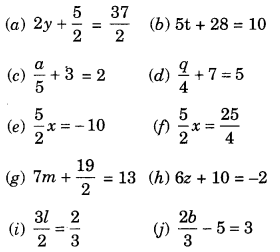Solution: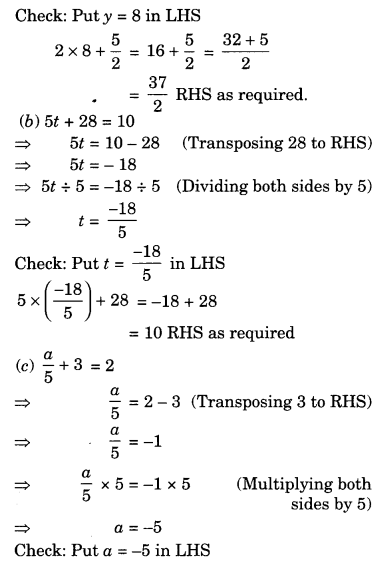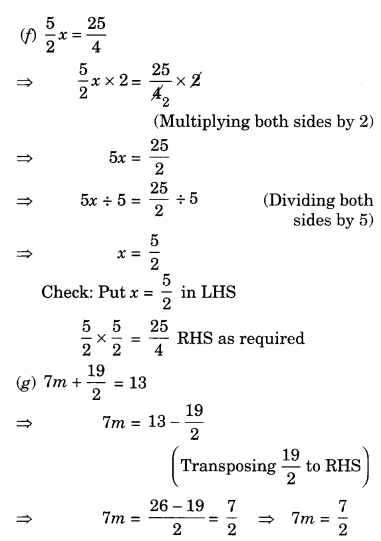Ex 4.3 Class 7 Maths Question 2.
Solve the following equations:
(а) 2(x + 4) = 12
(b) 3(n – 5) = 21
(c) 3(n – 5) = -21
(d) -4(2 + x) = 8
(e) 4(2 – x) = 8
Solution:
(a) 2(x + 4) = 12
⇒ $$\frac{2(x+4)}{2}=\frac{12}{2}$$ (Dividing both sides by 2)
⇒ x + 4 = 6
⇒ x = 6 – 4 (Transposing 4 to RHS)
⇒ x – 2
Check: Put x = 2 in LHS
2(2 + 4) = 2 × 6 = 12 RHS as required

(b) 3(n – 5) = 21
⇒ $$\frac{3(n-5)}{3}=\frac{21}{3}$$ (Dividing both sides by 3)
⇒ n – 5 = 7
⇒ n = 7 + 5 (Transposing 5 to RHS)
n = 12
Check: Put n = 12 in LHS
3(12 – 5) = 3 × 7 = 21 RHS as required

(c) 3(n – 5) = -21
⇒ $$\frac{3(n-5)}{3}=\frac{-21}{3}$$ (Dividing both sides by 3)
⇒ n – 5 = -7
⇒ n = -7 + 5 (Transposing 5 to RHS)
⇒ n = -2
Check: Put n = -2 in LHS
3(-2 – 5) = 3 × -7
= -21 RHS as required

(d) -4(2 + x) = 8
⇒ $$\frac{-4(2+x)}{-4}=\frac{8}{-4}$$ (Dividing both sides by -4)
⇒ 2 + x = -2
⇒ x = -2 – 2 (Transposing 2 to RHS)
⇒ x = —4
Check: Put x = -4 in LHS
-4(2 – 4) = -4 × -2 = 8 RHS as required

(e) 4(2-x) = 8
⇒ $$\frac{4(2-x)}{4}=\frac{8}{4}$$ (Dividing both sides by 4)
⇒ 2 – x = 2 – 2 (Transposing 2 to RHS)
⇒ -x = 0
∴ x = 0 (Multiplying both sides by -1)
Check: Put x = 0 in LHS
4(2 – 0) = 4 × 2 = 8 RHS as required

Ex 4.3 Class 7 Maths Question 3.
Solve the following equations:
(a) 4 = 5(p- 2)
(b) -4 = 5(p – 2)
(c) 16 = 4 + 3 (t + 2)
(d) 4 + 5(p – 1) = 34
(e) 0 = 16 + 4(m – 6)
Solution: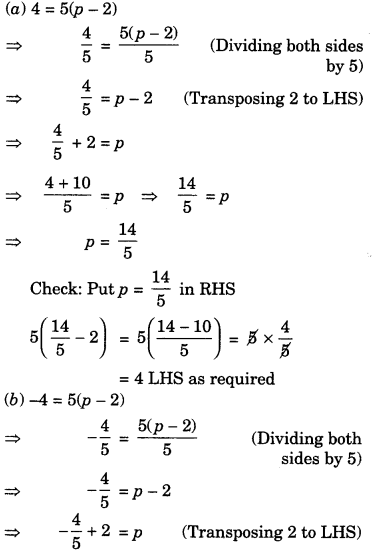(c) 16 = 4 + 3 (t + 2)
⇒ 16 – 4 = 3(t + 2) (Transposing 4 to LHS)
⇒ 12 = 3 (t + 2)
⇒ $$\frac{12}{3}=\frac{3(t+2)}{3}$$ (Dividing both sides by 3)
⇒ 4 = t + 2
⇒ 4 – 2 = t (Transposing 2 to LHS)
⇒ 2 = t or t = 2
Check: Put t = 2 in RHS
4 + 3(2 + 2) = 4 + 3 × 4 = 4 + 12
= 16 LHS as required

(d) 4 + 5(p – 1) = 34
⇒ 5(p – 1) = 34 – 4(Transposing 4 to RHS)
⇒ 5(p – 1) = 30
⇒ $$\frac{5(p-1)}{5}=\frac{30}{5}$$ (Dividing both sides by 5)
⇒ p – 1 = 6
⇒ P = 7
Check: Put p = 7 in LHS
4 + 5(7 – 1) = 4 + 5 × 6
= 4 + 30 = 34 RHS as required

(e) 0 = 16 + 4(m – 6)
⇒ 0 — 16 = 4(m – 6) (Transposing 16 to LHS)
⇒ -16 = 4(m – 6)
⇒ $$-\frac{16}{4}=\frac{4(m-6)}{4}$$ (Dividing both sides by 4)
⇒ -4 = m – 6
⇒ -4 + 6 = m (Transposing 6 to LHS)
⇒ 2 = m
or m = 2
Check: Put m = 2 in RHS
16 + 4(2 – 6) = 16 + 4 × (-4) = 16 – 16 = 0 LHS as required

Ex 4.3 Class 7 Maths Question 4.
(a) Construct 3 equations starting with x = 2
(b) Construct 3 equations starting with x – -2.
Solution:
(a) Possible equations are:
10x + 2 = 22; $$\frac{x}{5}=\frac{2}{5}$$ ; 5x – 3 = 7
(b) Possible equations are:
3x= -6; 3x + 7 = 1; 3x + 10 = 4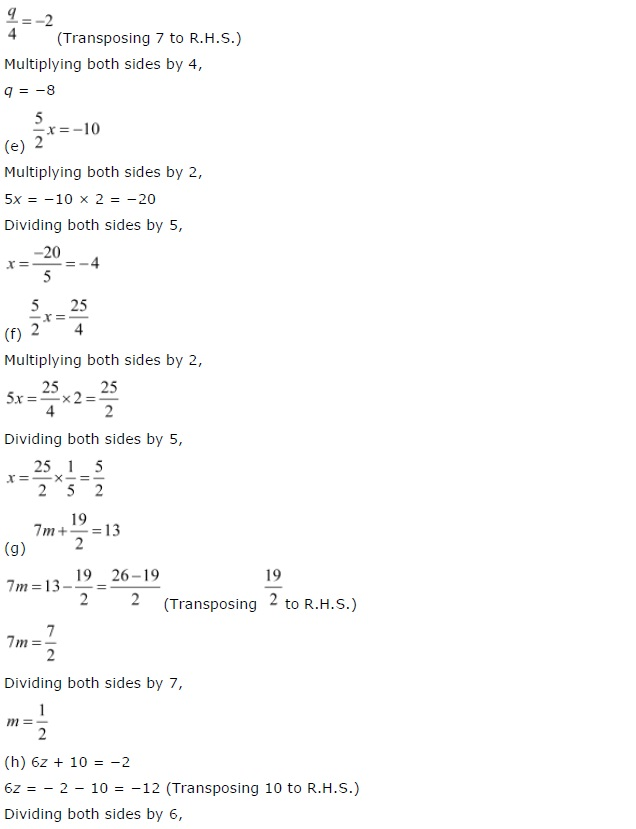+